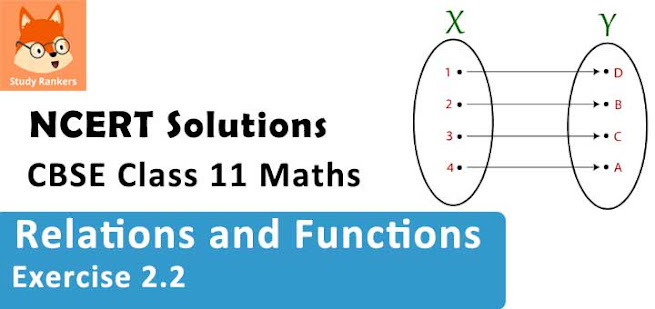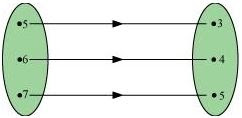# Class 11 Maths NCERT Solutions for Chapter 2 Relations and Functions Exercise 2.2### Relations and Functions Exercise 2.2 Solutions

1. Let A = {1, 2, 3, … , 14}. Define a relation R from A to A by R = {(x, y): 3x – y = 0, where x, y ∈ A}. Write down its domain, codomain and range.

Solution

The relation R from A to A is given as
R = {(xy): 3x – y = 0, where xy ∈ A}
i.e., R = {(xy): 3x = y, where xy ∈ A}
∴R = {(1, 3), (2, 6), (3, 9), (4, 12)}
The domain of R is the set of all first elements of the ordered pairs in the relation.
∴Domain of R = {1, 2, 3, 4}
The whole set A is the codomain of the relation R.
∴Codomain of R = A = {1, 2, 3, …, 14}
The range of R is the set of all second elements of the ordered pairs in the relation.
∴Range of R = {3, 6, 9, 12}

2. Define a relation R on the set N of natural numbers by R = {(xy): y = x + 5, x is a natural number less than 4; xy ∈ N}. Depict this relationship using roster form. Write down the domain and the range.

Solution

R = {(xy): y = x + 5, x is a natural number less than 4, xy ∈ N}
The natural numbers less than 4 are 1, 2, and 3.
∴R = {(1, 6), (2, 7), (3, 8)}
The domain of R is the set of all first elements of the ordered pairs in the relation.
∴ Domain of R = {1, 2, 3}
The range of R is the set of all second elements of the ordered pairs in the relation.
∴ Range of R = {6, 7, 8}

3. A = {1, 2, 3, 5} and B = {4, 6, 9}. Define a relation R from A to B by R = {(xy): the difference between x and y is odd; x ∈ A, ∈ B}. Write R in roster form.

Solution

A = {1, 2, 3, 5} and B = {4, 6, 9}
R = {(xy): the difference between x and y is odd; x ∈ A, ∈ B}
∴R = {(1, 4), (1, 6), (2, 9), (3, 4), (3, 6), (5, 4), (5, 6)}

4. The given figure shows a relationship between the sets P and Q. write this relation
(i) in set-builder form (ii) in roster form.
What is its domain and range?Solution

According to the given figure, P = {5, 6, 7}, Q = {3, 4, 5}
(i) R = {(x, y): y = x – 2; x ∈ P} or R = {(x, y): y = x – 2 for x = 5, 6, 7}

(ii) R = {(5, 3), (6, 4), (7, 5)}
Domain of R = {5, 6, 7}
Range of R = {3, 4, 5}

5. Let A = {1, 2, 3, 4, 6}. Let R be the relation on A defined by
{(ab): ab ∈ A, b is exactly divisible by a}.
(i) Write R in roster form
(ii) Find the domain of R
(iii) Find the range of R.

Solution

A = {1, 2, 3, 4, 6}, R = {(ab): ab ∈ A, b is exactly divisible by a}
(i) R = {(1, 1), (1, 2), (1, 3), (1, 4), (1, 6), (2, 2), (2, 4), (2, 6), (3, 3), (3, 6), (4, 4), (6, 6)}
(ii) Domain of R = {1, 2, 3, 4, 6}
(iii) Range of R = {1, 2, 3, 4, 6}

6. Determine the domain and range of the relation R defined by R = {(xx + 5): x ∈ {0, 1, 2, 3, 4, 5}}.

Solution

R = {(xx + 5): x ∈ {0, 1, 2, 3, 4, 5}}
∴ R = {(0, 5), (1, 6), (2, 7), (3, 8), (4, 9), (5, 10)}
∴Domain of R = {0, 1, 2, 3, 4, 5}
Range of R = {5, 6, 7, 8, 9, 10}

7. Write the relation R = {(xx3): is a prime number less than 10} in roster form.

Solution

R = {(xx3): is a prime number less than 10}
The prime numbers less than 10 are 2, 3, 5, and 7.
∴R = {(2, 8), (3, 27), (5, 125), (7, 343)}

8. Let A = {xy, z} and B = {1, 2}. Find the number of relations from A to B.

Solution

It is given that A = {xy, z} and B = {1, 2}.
∴ A × B = {(x, 1), (x, 2), (y, 1), (y, 2), (z, 1), (z, 2)}
Since n(A × B) = 6, the number of subsets of A × B is 26.
Therefore, the number of relations from A to B is 26.

9. Let R be the relation on Z defined by R = {(ab): ab ∈ Z, – b is an integer}. Find the domain and range of R.

Solution

R = {(ab): ab ∈ Z, – b is an integer}
It is known that the difference between any two integers is always an integer.
∴Domain of R = Z
Range of R = Z